#### 期刊菜单

Underwater Image Enhancement Based on 2D Cubic Spline Wavelet and Red Channel Prior
DOI: 10.12677/JISP.2023.121006, PDF, HTML, XML, 下载: 34  浏览: 88  国家自然科学基金支持

Abstract: To address the problems of low contrast, blurred details and color distortion that occur in underwater images, this paper proposes a two-dimensional cubic spline wavelet with a dark channel prior for underwater image enhancement. The underwater image is removed from the fog blur using the red channel a priori method and then normalized for white balance. The original underwater image is also subjected to additive wavelet decomposition using two-dimensional cubic spline wavelets to produce low-frequency and high-frequency sub-images. The high-frequency sub-images coefficients are scaled up to enhance the detail information. Finally, the processed high-frequency sub-images and the white-balanced image are summed to obtain the enhanced image. The experimental results show that the algorithm in this paper can effectively eliminate the image color distortion and the enhanced image presents high contrast and clear details. It offers significant improvements in contrast, color, edge retention and naturalness compared to the typical methods related to current underwater image enhancement.

1. 引言

2. 相关知识

2.1. 不可分加性小波

${p}_{0}$ 为原图像，则有：

$\begin{array}{l}{H}_{0}\left({p}_{0}\right)={p}_{1},{w}_{1}={p}_{0}-{p}_{1},\\ {H}_{0}\left({p}_{1}\right)={p}_{2},{w}_{2}={p}_{1}-{p}_{2},\\ {H}_{0}\left({p}_{2}\right)={p}_{3},{w}_{1}={p}_{2}-{p}_{3},\end{array}$ (1)

……

${p}_{0}=\underset{l=1}{\overset{n}{\sum }}{w}_{l}+{p}_{r}$ (2)

2.2. 红通道先验

$\begin{array}{l}1-{I}^{r}\left(x,y\right)=t\left(x,y\right)\left(1-{J}^{r}\left(x,y\right)\right)+\left(1-t\left(x,y\right)\right)\left(1-{A}^{r}\right)\\ {I}^{g}\left(x,y\right)=t\left(x,y\right){J}^{g}\left(x,y\right)+\left(1-t\left(x,y\right)\right){A}^{g}\\ {I}^{b}\left(x,y\right)=t\left(x,y\right){J}^{b}\left(x,y\right)+\left(1-t\left(x,y\right)\right){A}^{b}\end{array}$ (3)

$\stackrel{\to }{I}=\left({I}^{r},{I}^{g},{I}^{b}\right)$$\stackrel{\to }{J}=\left({J}^{r},{J}^{g},{J}^{b}\right)$ 分别表示原始图像和增强后的水下图像， $t\left(x,y\right)$ 表示水下环境中介质透射率，A表示水下环境中光照强度。根据暗通道先验方法，将改进的暗通道表示为：

${J}^{red}\left(x,y\right)=\mathrm{min}\left(\underset{\left({x}^{\prime },{y}^{\prime }\right)\in \Omega \left(x,y\right)}{\mathrm{min}}\left(1-{J}^{r}\left({x}^{\prime },{y}^{\prime }\right)\right),\underset{\left({x}^{\prime },{y}^{\prime }\right)\in \Omega \left(x,y\right)}{\mathrm{min}}\left(1-{J}^{g}\left({x}^{\prime },{y}^{\prime }\right)\right),\underset{\left({x}^{\prime },{y}^{\prime }\right)\in \Omega \left(x,y\right)}{\mathrm{min}}\left(1-{J}^{b}\left({x}^{\prime },{y}^{\prime }\right)\right)\right)$ (4)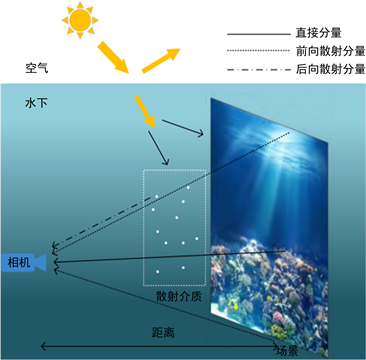Figure 1. Underwater models

2.3. 灰度世界算法

$Baver=Gaver=Raver$ (5)

$\begin{array}{l}{R}_{avg}=\left(\underset{i=1}{\overset{M}{\sum }}\underset{j=1}{\overset{N}{\sum }}{R}_{ij}\right)/\left(M\ast N\right)\\ {G}_{avg}=\left(\underset{i=1}{\overset{M}{\sum }}\underset{j=1}{\overset{N}{\sum }}{G}_{ij}\right)/\left(M\ast N\right)\\ {B}_{avg}=\left(\underset{i=1}{\overset{M}{\sum }}\underset{j=1}{\overset{N}{\sum }}{B}_{ij}\right)/\left(M\ast N\right)\end{array}$ (6)

${R}_{gain}=Gray/{R}_{avg},{G}_{gain}=Gray/{G}_{avg},{B}_{gain}=Gray/{B}_{avg}$ (7)

$\begin{array}{l}{R}_{out}={R}_{in}\ast {R}_{gain}\\ {G}_{out}={G}_{in}\ast {G}_{gain}\\ {B}_{out}={B}_{in}\ast {B}_{gain}\end{array}$ (8)

3. 基于不可分加性小波与暗通道先验的水下图像增强方法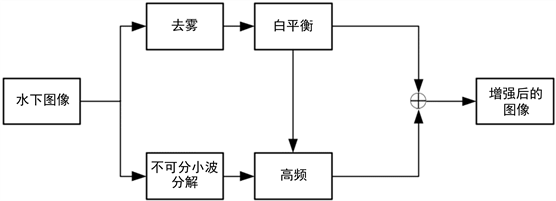Figure 2. Algorithm processing flow in this paper

${H}_{0}=\frac{1}{256}\left(\begin{array}{ccccc}1& 4& 6& 4& 1\\ 4& 16& 24& 16& 4\\ 6& 24& 36& 24& 6\\ 4& 16& 24& 16& 4\\ 1& 4& 6& 4& 1\end{array}\right)$ (9)

$\stackrel{^}{A}\left(l,m\right)=\frac{G\left(l,m\right)}{J\left(l,m\right)}A\left(l,m\right)$，对所有的 $l,m$ 进行处理 (10)

$M\left(l,m\right)=G\left(l,m\right)+\stackrel{^}{A}\left(l,m\right)$，对所有的 $l,m$ 进行处理 (11)

4. 实验结果与分析

4.1. 实验数据与环境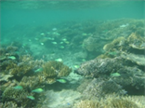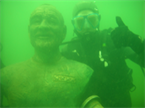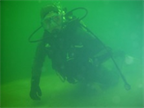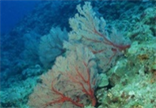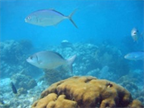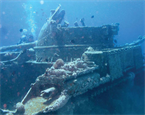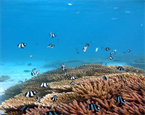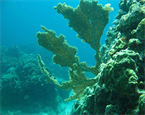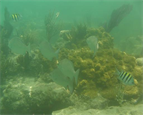Figure 3. Underwater images used for experiments

4.2. 实验结果对比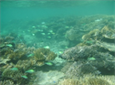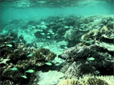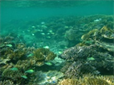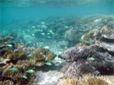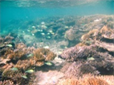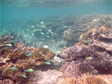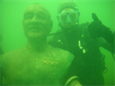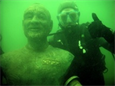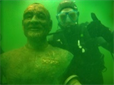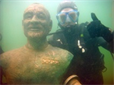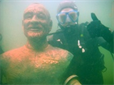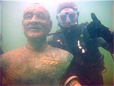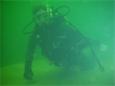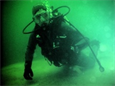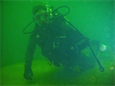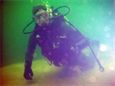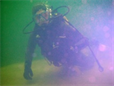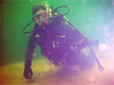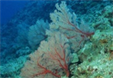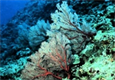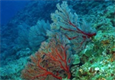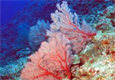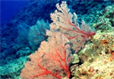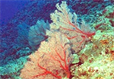原始图像4 HE方法 DCP方法 RCP方法 NUDCP方法 本文方法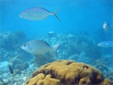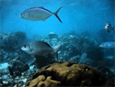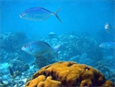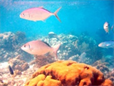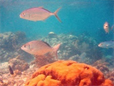原始图像5 HE方法 DCP方法 RCP方法 NUDCP方法 本文方法

Figure 4. Comparison of underwater image enhancement methods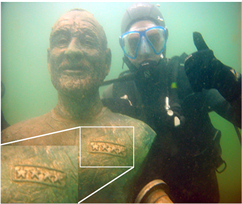(a) RCP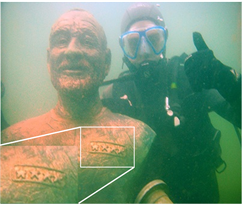(b) NUDCP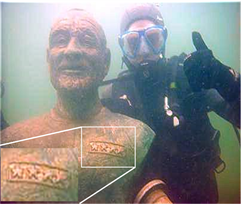(c) 本文方法

Figure 5. Comparison of the details of the enhancement results of RCP method (a), NUDCP method (b) and this paper’s method (c)

$\text{PCQI}\left(a,b\right)={q}_{i}\left(a,b\right)\cdot {q}_{c}\left(a,b\right)\cdot {q}_{s}\left(a,b\right)$ (12)

$\text{Sharpness}=\sum {\left(I\left(x+2,y\right)-I\left(x,y\right)\right)}^{2}+{\left(I\left(x,y+2\right)-I\left(x,y\right)\right)}^{2}$ (13)Table 1. Evaluation index of underwater image enhancement results

5. 结束语

  李黎, 王惠刚, 刘星. 基于改进暗原色先验和颜色校正的水下图像增强[J]. 光学学报, 2017, 37(12): 168-176.  Liang, Z., Wang, Y., Ding, X., Mi, Z. and Fu, X. (2021) Single Underwater Image Enhancement by Attenuation Map Guided Color Correction and Detail Preserved Dehazing. Neurocomputing, 425, 160-172. https://doi.org/10.1016/j.neucom.2020.03.091  郭继昌, 岳惠惠, 张怡, 刘迪, 刘晓雯, 郑司达. 图像增强对显著性目标检测的影响研究[J]. 中国图象图形学报, 2022, 27(7): 2129-2147.  Arici, T., Dikbas, S. and Altunbasak, Y. (2009) A Histogram Modification Framework and Its Application for Image Contrast Enhancement. IEEE Transactions on Image Processing, 18, 1921-1935. https://doi.org/10.1109/TIP.2009.2021548  Chang, Y.C. and Chang, C.M. (2010) A Simple Histogram Modification Scheme for Contrast Enhancement. IEEE Transactions on Consumer Electronics, 56, 737-742. https://doi.org/10.1109/TCE.2010.5505995  董丽丽, 丁畅, 许文海. 基于直方图均衡化图像增强的两种改进方法[J]. 电子学报, 2018, 46(10): 2367-2375.  Chen, Y., Feng, P., Yang, J. and XIE, Z. (2012) An Adaptive Enhancement Algorithm of Low Illumine Color Video Image. International Journal of Sensing, Computing & Control, 2, 79-86.  仲伟峰, 袁东雪. 基于低照度的有雾彩色图像增强算法[J]. 激光与光电子学进展, 2020, 57(16): 223-229.  黄仁, 梁笑. 基于多尺度子带划分的雾霾天气图像增强方法[J]. 计算机应用, 2014, 34(S1): 215-218.  方帅, 杨静荣, 曹洋, 等. 图像引导滤波的局部多尺度Retinex算法[J]. 中国图象图形学报, 2012, 17(7): 748-755.  Li, Q. and Liu, Q. (2015) Adaptive Enhancement Algorithm for Low Illumination Images Based on Wavelet Transform. Chinese Journal of Lasers, 42, 272-278. https://doi.org/10.3788/CJL201542.0209001  Chiang, J.Y. and Chen, Y.C. (2011) Underwater Image Enhancement by Wavelength Compensation and Dehazing. IEEE Transactions on Image Processing, 21, 1756-1769. https://doi.org/10.1109/TIP.2011.2179666  Abd-Al Ameer, Z.S., Daway, H.G. and Kareem, H.H. (2019) Enhancement Underwater Image Using Histogram Equalization Based on. Journal of Engineering and Applied Sciences, 14, 641-647. https://doi.org/10.36478/jeasci.2019.641.647  Sun, X., Liu, L., Li, Q., Dong, J., Lima, E. and Yin, R. (2019) Deep Pixel-to-Pixel Network for Underwater Image Enhancement and Restoration. IET Image Processing, 13, 469-474. https://doi.org/10.1049/iet-ipr.2018.5237  Patil, P., Patil, C., Patil, U. and Dmello, S. (2019) Enhancing Underwater Image by Fusion. International Journal of Computer Science and Mobile Computing, 8, 69-72.  Islam, M. J., Xia, Y. and Sattar, J. (2020) Fast Underwater Image Enhancement for Improved Visual Perception. IEEE Robotics and Automation Letters, 5, 3227-3234. https://doi.org/10.1109/LRA.2020.2974710  Zhang, W., Li, G. and Ying, Z. (2018) Underwater Image Enhancement by the Combination of Dehazing and Color Correction. Pacific Rim Conference on Multimedia, Hefei, 21-22 September 2018, 145-155. https://doi.org/10.1007/978-3-030-00764-5_14  Mi, Z., Liang, Z., Wang, Y., Fu, X. and Chen, Z. (2018) Multi-Scale Gradient Domain Underwater Image Enhancement. 2018 OCEANS-MTS/IEEE Kobe Techno-Oceans (OTO), Kobe, 28-31 May 2018, 1-5. https://doi.org/10.1109/OCEANSKOBE.2018.8559180  Moni, J. and Prakash, A.J. (2018) Color Balance and Fusion for Underwater Image Enhancement: Survey. International Research Journal of Engineering and Technology (IRJET), 5, 1391-1394.  Cheng, N., Zhao, T., Chen, Z. and Fu, X. (2018) Enhancement of Underwater Images by Super-Resolution Generative Adversarial Networks. Proceedings of the 10th International Conference on Internet Multimedia Computing and Service, Nanjing, 17-19 August 2018, 1-4. https://doi.org/10.1145/3240876.3240881  Hou, M., Liu, R., Fan, X. and Luo, Z. (2018) Joint Residual Learning for Underwater Image Enhancement. 2018 25th IEEE International Conference on Image Processing (ICIP), Athens, 7-10 October 2018, 4043-4047. https://doi.org/10.1109/ICIP.2018.8451209  AncutiT, C. O., Ancuti, C. andBaltaT, H. (2018) Effective Local Backscattering Estimation for Underwater Image Enhancement. OCEANS 2018 MTS/IEEE Charleston, Charleston, 22-25 October 2018, 1-4. https://doi.org/10.1109/OCEANS.2018.8604731  Deperlioglu, O. and Kose, U. (2018) Practical Method for the Underwater Image Enhancement with Adjusted CLAHE. 2018 International Conference on Artificial Intelligence and Data Processing (IDAP), Malatya, 28-30 September 2018, 1-6. https://doi.org/10.1109/IDAP.2018.8620727  Mathur, M. and Goel, N. (2018) Enhancement of Underwater Images Using White Balancing and Rayleigh-Stretching. 2018 5th International Conference on Signal Processing and Integrated Networks (SPIN), Noida, 22-23 February 2018, 924-929. https://doi.org/10.1109/SPIN.2018.8474042  Chen, Y.K., Miaou, S.G., Lin, Y.C. and Chen, Y.M. (2018) Color Image Enhancement Using Luminance Histogram Equalization and Two-Factor Saturation Control. 2018 Joint 7th International Conference on Informatics, Electronics & Vision (ICIEV) and 2018 2nd International Conference on Imaging, Vision & Pattern Recognition (icIVPR), Kitakyushu, 25-29 June 2018, 159-164. https://doi.org/10.1109/ICIEV.2018.8641063  Nunez, J., Otazu, X., Fors, O., Prades, A., Pala, V. and Arbiol, R. (1999) Multiresolution-Based Image Fusion with Additive Wavelet Decomposition. IEEE Transactions on Geoscience and Remote Sensing, 37, 1204-1211. https://doi.org/10.1109/36.763274  Jaffe, J.S. (1990) Computer Modeling and the Design of Optimal Underwater Imaging Systems. IEEE Journal of Oceanic Engineering, 15, 101-111. https://doi.org/10.1109/48.50695  Ketcham, D.J. (1976) Real-Time Image Enhancement Techniques. Proceedings of SPIE-OSA, 74, 120-125. https://doi.org/10.1117/12.954708  He, K., Sun, J. and Tang, X. (2010) Single Image Haze Removal Using Dark Channel Prior. IEEE Transactions on Pattern Analysis and Machine Intelligence, 33, 2341-2353. https://doi.org/10.1109/TPAMI.2010.168  Galdran, A., Pardo, D., Picón, A. and Alvarez-Gila, A. (2015) Automatic Red-Channel Underwater Image Restoration. Journal of Visual Communication and Image Representation, 26, 132-145. https://doi.org/10.1016/j.jvcir.2014.11.006  Song, W., Wang, Y., Huang, D., Liotta, A. and Perra, C. (2020) Enhancement of Underwater Images with Statistical Model of Background Light and Optimization of Transmission Map. IEEE Transactions on Broadcasting, 66, 153-169. https://doi.org/10.1109/TBC.2019.2960942  Wang, S., Ma, K., Yeganeh, H., Wang, Z. and Lin, W. (2015) A Patch-Structure Representation Method for Quality Assessment of Contrast Changed Images. IEEE Signal Processing Letters, 22, 2387-2390. https://doi.org/10.1109/LSP.2015.2487369  Treeby, B.E., Varslot, T.K., Zhang, E.Z., Laufer, J.G. and Beard, P.C. (2011) Automatic Sound Speed Selection in Photoacoustic Image Reconstruction Using an Autofocus Approach. Journal of Biomedical Optics, 16, Article ID: 090501. https://doi.org/10.1117/1.3619139